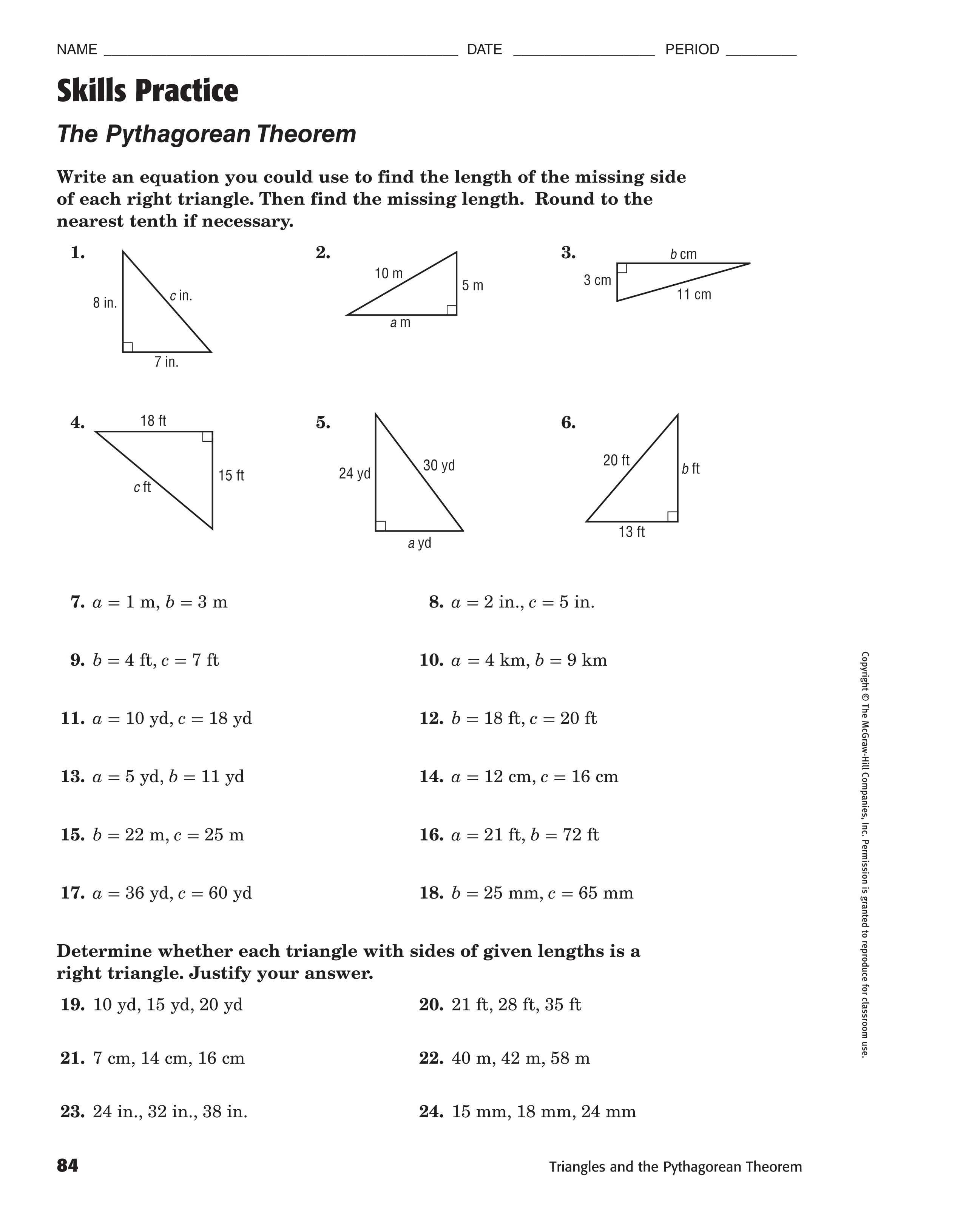Pythagorean Theorem (Skills Practice - Odd)
starstarstarstarstarstarstarstarstarstar
by Reginald Shorter
| 10 Questions1
2
3
4
5
6
7
8
9
10
1
1 pt
Write an equation you could use to find the length of the missing side
of each right triangle. Then find the missing length. Round to the
nearest tenth if necessary.
2
1 pt
Write an equation you could use to find the length of the missing side
of each right triangle. Then find the missing length. Round to the
nearest tenth if necessary.
3
1 pt
Write an equation you could use to find the length of the missing side
of each right triangle. Then find the missing length. Round to the
nearest tenth if necessary.
4
1 pt
Write an equation you could use to find the length of the missing side
of each right triangle. Then find the missing length. Round to the
nearest tenth if necessary.
5
1 pt
Write an equation you could use to find the length of the missing side
of each right triangle. Then find the missing length. Round to the
nearest tenth if necessary.
6
1 pt
Write an equation you could use to find the length of the missing side
of each right triangle. Then find the missing length. Round to the
nearest tenth if necessary.
7
1 pt
Write an equation you could use to find the length of the missing side
of each right triangle. Then find the missing length. Round to the
nearest tenth if necessary.
8
1 pt
Write an equation you could use to find the length of the missing side
of each right triangle. Then find the missing length. Round to the
nearest tenth if necessary.
9
1 pt
Write an equation you could use to find the length of the missing side
of each right triangle. Then find the missing length. Round to the
nearest tenth if necessary.
10
1 pt
Write an equation you could use to find the length of the missing side
of each right triangle. Then find the missing length. Round to the
nearest tenth if necessary.
Add to my formatives list